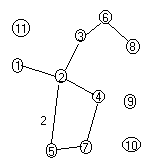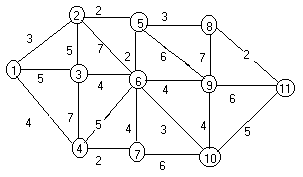Erich Prisner
UMUC SG
Spring 2002

## Floyd's Algorithm

See the adjacency matrix of one of the graphs shown by clicking on the corresponding graph button. Click 11 times on the "increase m" button to see the distance matrix --- "-" denotes infinity. Again Am denotes the distance matrix with only paths with intermediate vertices in 1,2,...m allowed. We use the formula
am(i,j) = min{am-1(i,j),am-1(i,m)+am-1(m,j)}m =